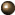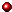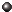# Distributed Snapshots: Determining Global States of Distributed Systems

K. Mani Chandy @ Texas-Austin, Leslie Lamport @ Stanford

ACM Trans. on Computer System 1985

## 1. IntroductionProblem: How to capture the global state of distributed system where only local states are availableLocal state: state of each process, state about msgs each process has sent or receivedChallenge: how to get the global snapshot from bunch of local snapshots taken not at the same time: maybe undecidable!What if the problem is reduced only to stable properties?Stable property y of system D = predicate y on state S of D s.t.if y(S) is true, then y(S') is always true for every S' which is reachable from Sexamples: termination of a computation, deadlock of the system, tokens disappeared, etc.Deadlock detection & termination detection problems are special case of stable property detection problem!Also many distributed algorithms are structured as a seq. of phases, s.t.Each phase = transient part + stable parttransient part: actual work of the phase is donestable part: system waits indefinitely, doing nothing, until certain stable property becomes trueMain attention of the paper: detection of the termination of a phase

## 2. Model of a Distributed SystemA distributed system = directed graph G(V, E)V = set of processes, E = set of channels (connections) between processes in VChannelinfinite buffer capacityreliable and in-order deliverydelay is indefinite but finitestate of the channel = the set of msgs injected to the channel but not received yetProcess = state transition machine(St, Ev, a)St = the set of statea = the initial stateEv = the set of eventAn event is an atomic action and may change the state of the process and possibly the state of channel incident to the processEvent = (p, s, s', M, c)p = the process in which the event occurss = the state of p before the events' = the state of p after the eventc(optional) = a channel incident to pM(optional) = a msg sent to or received from cState of distributed system = ( {process_state}, {channel_state}, init_state )init_state = each component process is in its init state & channels are emptyValid event = (p, s, s', M, c): an event which can occur on the current system statep is in s; if c is incident to (not from) p, then M is in the head of cValid seq. of event: obviousnext_state of the system = next (system_state, event): obviousA computation = a sequence of states of the system, starting from init_state

## 3. The Algorithm

### 3.1 Motivation for the Steps of the AlgorithmLocal state recodingProcess state: recorded by each processChannel state: processes connected by the channel should cooperateThe examples in this section show two anomalies of system states which are composed from local states taken at different timesAnomalies in these examples occur because:channel's state includes msgs sent after sender's state is recordedchannel's state is taken before sender's state: msgs sent in between the time when channel's state is taken and when sender's is taken are missedThe condition for consistent system recordThe state of channel should be the sequence of messages which aresent before sender's state is recordednot received when the receiver's state is recordedIdeasender records its state -> sends 'mark' msg ->receiver records its state ->receiver records channel state until it receives the 'mark' msg

### 3.2 Global-State-Detection Algorithm OutlineMarker-Sending Rule:for each outbound channel csend 'marker' after recoding its state and before sending any further messageMarker-Receiving Rule

if I have not recorded my state {

record my state with current status;

record channel state as 'empty'

} else {

record channel state as the seq. of msgs received after I recorded my state and before I got the 'mark' msg

}In short, each process records its own state and states of incoming channels!

### 3.3 Termination of the Global State Recoding AlgorithmReceiving a marker make the receiver record its state!Periodic recording by all processes guarantees the terminationIf the graph is strongly connected, single process spontaneously recording its state guarantees the termination of the algorithmHow to collect those local states to combine the global state is described in another paper

## 4. Properties of the Recorded Global StateNotation: S1 ->(e1)-> S2 = global state S1 goes to S2 by e1. Hence S1, ... Sn is equivalent to e1, ..., en.Recording local states (including propagating marker) takes time -> System keeps changing its real state while the recording is going on=> Recorded global state can be different from the state at time of record reading. Even worse,if system goes S1, S2, ..., Si, ..., Sj, ..., Sn, and the recording starts at Si and terminates at Sj,the recorded state S* can be different from any of Si, ..., SjHowever, it is proved that:S* is reachable from Si, andSj is reachable from S*Theorem: There exists a computation e'1, e'2, ..., e'n, s.t.e1, ..., ei-1 = e'1, ..., e'i-1 & ej, ..., en = e'j, ..., e'ne'i, ..., e'j-1 is a permutation of ei, ..., ej-1for i >= x, or x >= j, Sx = S'xthere exists k, s.t., i <= k <= j, S* = S'k

## 5. Stability DetectionInput: stable property y, i.e., a predicateOutput: Boolean variable definite s.t.,(y(initial state of the system) -> definite) && (definite -> y(final state of the system))Note:

 y (init state) definite y (init state) -> definite F T/F T T T T T F F
 definite y (final state) definite -> y (final state) F T/F T T T T T F FHence, definite = false means that y(initial state) = false, and definite = true means that y(final state) = trueAlgorithm is obvious from the definition of stabilityrecord a global state S*;definite = y(S*);